## RS Aggarwal Class 7 Solutions Chapter 12 Simple Interest Ex 12A

These Solutions are part of RS Aggarwal Solutions Class 7. Here we have given RS Aggarwal Solutions Class 7 Chapter 12 Simple Interest Ex 12A.

Other Exercises

Find the simple interest and the amount when :
Question 1.
Solution:
Principal (P) = Rs. 6400
Rate (r) = 6% p.a.
Time (t) = 2 yearsQuestion 2.
Solution:
Principal (P) = Rs. 2650
Rate (r) = 8% p.a.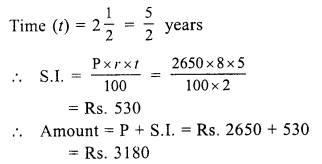Question 3.
Solution:
Principal (P) = Rs. 1500
Rate (r) = 12% p.a.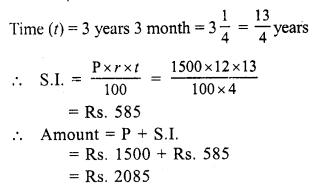Question 4.
Solution:
Principal (P) = Rs. 9600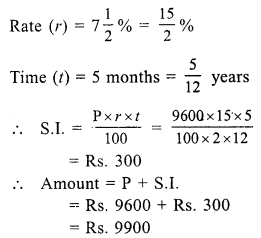Question 5.
Solution:
Principal (P) = Rs. 5000
Rate (r) = 9% p.a.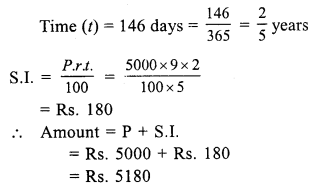Find the time when :
Question 6.
Solution:
Principal (P) = Rs. 6400
S.I. = Rs. 1152
Rate (r) = 6% p.a.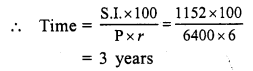Question 7.
Solution:
Principal (P) = Rs. 9540
S.I. = Rs. 1908
Rate (r) = 8% p.a.Question 8.
Solution:
Amount (A) = Rs. 6450
Principal (P) = Rs. 5000
S.I. = A – P = Rs. (6450 – 5000) = Rs. 1450
Rate (r) = 12% p.a.Find the rate when :
Question 9.
Solution:
Principal (P) = Rs. 8250
S.I. = Rs. 1100
Time (t) = 2 years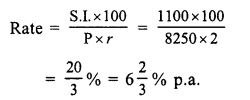Question 10.
Solution:
Principal (P) = Rs. 5200
S.I. = Rs. 975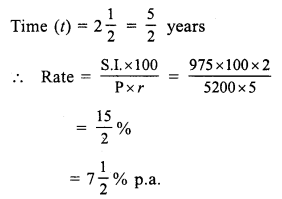Question 11.
Solution:
Principal (P) = Rs. 3560
Amount (A) = Rs. 4521.20
S.I. = A – P = Rs. 4521.20 – 3560 = Rs. 961.20
Time (t) = 3 years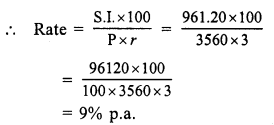Question 12.
Solution:
Principal (P) = Rs. 6000
Rate (r) = 12% p.a.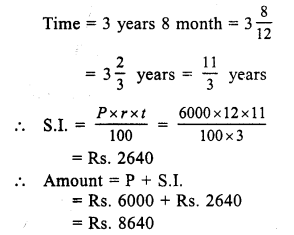Question 13.
Solution:
Principal = Rs. 12600
Rate (A) = 15% p.a.
Time (t) = 3 yearsAmount = P + S.I. = Rs. 12600 + Rs. 5670 = Rs. 18270
Amount paid in cash = Rs. 7070
Balance = Rs. 18270 – 7070 = Rs. 11200
Price of goat = Rs. 11200

Question 14.
Solution:
S.I. = Rs. 829.50
Rate (r) = 10% p.a.
Time (t) = 3 years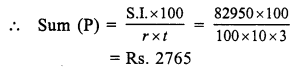Question 15.
Solution:
Amount (A) = Rs. 3920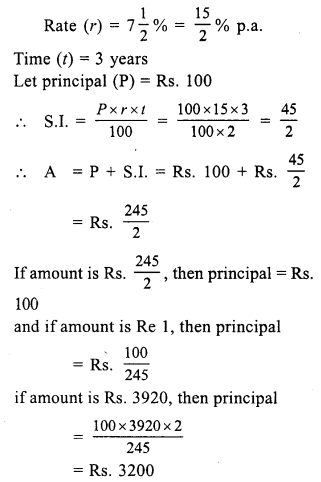Question 16.
Solution:
Amount = Rs. 4491
Let principal (P) = Rs. 100
Rate (r) =11% p.a.
Time (t) = 2 years 3 months = 2$$\frac { 1 }{ 4 }$$ = $$\frac { 9 }{ 4 }$$ years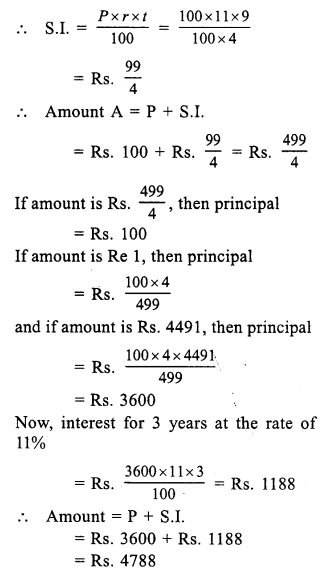Question 17.
Solution:
Amount = Rs. 12122
Let principal (P) = Rs. 100
Rate (r) = 8% p.a.
Time (t) = 2 years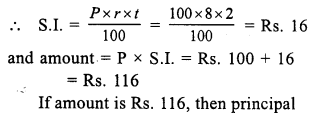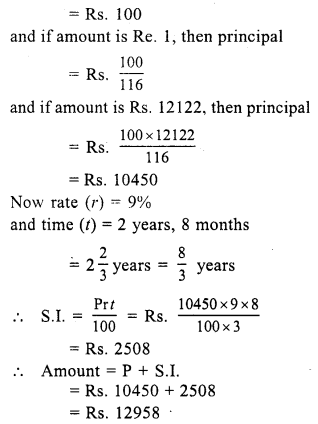Question 18.
Solution:
Amount (A) = Rs. 4734
Principal (P) = Rs. 3600
S.I. = A – P = Rs. 4734 – Rs. 3600 = Rs. 1134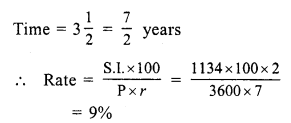Question 19.
Solution:
In first case,
Amount (A) = Rs. 768
Principal (P) = Rs. 640
S.I. = A – P = Rs. 768 – 640 = Rs. 128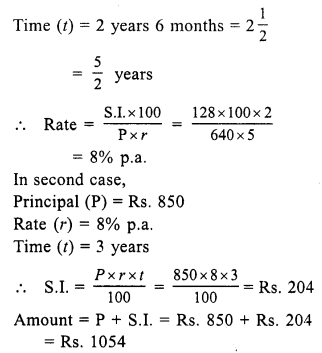Question 20.
Solution:
Principal (P) = Rs. 5600
Amount (A) = Rs. 6720
S.I. = A – P = Rs. 6720 – 5600 = Rs. 1120
Rate (r) = 8% p.a.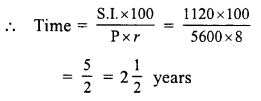Question 21.
Solution:
Let principal (P) = Rs. 100
then amount (A) = Rs. 100 x $$\frac { 8 }{ 5 }$$ = Rs. 160
S.I. = A – P = Rs. 160 – 100 = Rs. 60
Time (t) = 5 yearsQuestion 22.
Solution:
Amount in 3 years = Rs. 837
Amount in 2 years = Rs. 783
Difference = Rs. 837 – Rs. 783 = Rs. 54
Rs. 54 is interest for 1 year
Interest for 2 years = 2 x 54 = Rs. 108
Principal = Rs. 783 – 108 = Rs. 675Question 23.
Solution:
Amount in 5 years = Rs. 5475
Amount in 3 years = Rs. 4745
Interest for 2 years = Rs. 5475 – 4745 = Rs. 730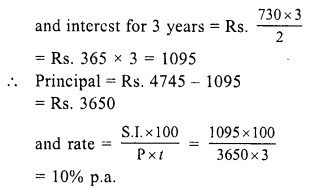Question 24.
Solution:
Total sum = Rs. 3000
Let first part = Rs. x
Then second part = Rs. (3000 – x)
Now, interest on first part at the rate ofQuestion 25.
Solution:
Total sum =Rs. 3600
Let first part = Rs. x
Then second part = Rs. (3600 – x)
Interest on first part for 1 year at 9% p.a.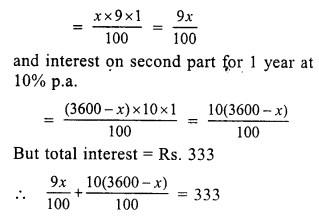⇒ 9x + 10 (3600 – x) = 33300
⇒ 9x + 36000 – 10x = 33300
⇒ -x = 33300 – 36000
⇒ -x = – 2700
⇒ x = 2700
First part = Rs. 2700
and second part = Rs. 3600 – 2700 = Rs. 900

Hope given RS Aggarwal Solutions Class 7 Chapter 12 Simple Interest Ex 12A are helpful to complete your math homework.

If you have any doubts, please comment below. Learn Insta try to provide online math tutoring for you.